## ICSE Class 9 Physics Sample Question Paper 4 with Answers

Section-1
(Attempt all questions from this section)

Question 1.
(a) What do you understand by (i) amplitude and (ii) frequency of oscillation of a simple pendulum ?
(b) Draw a graph of l, the length of a simple pendulum, against T2, the square of its time period.
(c) Is a month equal to a lunar month ? Give reason.
(f) The iron bob of a given pendulum is replaced by a lead one of the same radius. Lead is known to be denser than iron. What would be the effect of this change on the pendulum’s time period ?
(g) An astronaut carries a second’s pendulum to the moon where the gravity is only 1/9th of that of the earth. What will be the time period of the pendulum there ?
(a) (i) The amplitude of a simple pendulum is the maximum displacement of its bob from its equilibrium or mean position.
(ii) The frequency of oscillation of simple pendulum equals the number of complete oscillation made by it in one second.

(b) The required graph is a straight line, e., T2 α l.(c) No, the month is defined in terms of the motion of the earth round the sun while the lunar month is defined in terms of the motion of the moon round the earth.

(d) Since the lead bob is of the same radius as the iron one, there is no change in the length of the pendulum. As time period is independent of the mass of the bob, there would be no effect on the time period of the pendulum.

(e) We haveQuestion 2.
(a) What physical quantity is represented by the symbol ‘g’ ? What is its meaning ?
(b) Fill in the blanks :
(c) The physical quantity representing rate of change of displacement is known as ………………………….
(d) The motion of a body for which the acceleration is zero is known as……………………….
(g) The electrons in a T.V. tube travel a distance of 0.2 m, at a constant speed, in 10-5 What is their average speed ?
(h) Comment on the type of motion represented by the velocity-time graph shown in the figure. What conclusion can you draw from this graph ?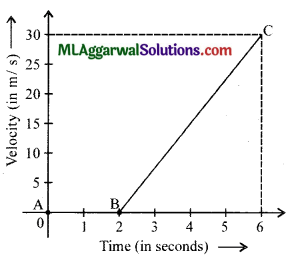(e) Rhea takes 15 minutes to go from her home to school and 20 minutes for her return journey. If the distance between the school and the home is 3.5 km, what is her (i) average speed, (ii) average velocity?
(a) The symbol ‘g’ represents the acceleration due to gravity. It is the value of acceleration, with which all freely falling objects fall towards the earth. The value of ‘g’ is nearly 9.8 ms-2 or nearly 980 cm s-2.

(b) (i) Velocity
(ii) Uniform motion.

(c) We know,
$$\text { Average speed }(v)=\frac{\text { Total distance travelled }}{\text { Total time taken }}$$
Here, distance travelled= 0.2 m, time taken = 10-5 s.
$$v=\frac{0.2}{10^{-5}}=2 \times 10^{4} \mathrm{~m} / \mathrm{s}$$(d) The particle, in this case, remains at rest (v – 0) from t = 0 to t = 2. After that, it has a uniformly accelerated motion.
The slope of the graph $$=\frac{30-0}{6-2}=\frac{30}{4}=7.5$$
This slope represents the acceleration. We can, therefore, conclude that the particle moves with a uniform acceleration of 7.5 ms-2 from f = 2s to f = 6s.

(e) (i)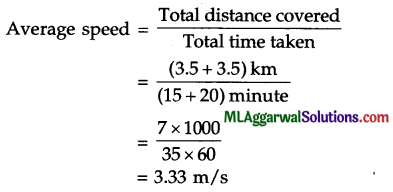(ii)The total displacement is zero because the starting and end points of the displacement of Rhea are the same (she is back home).

Question 3.
(a) A girl running on the ground, trips on a stone. What is likely to happen to her and why?
(b) The foundations of a high rise building are made very wide. Why?
(c) Will one kilogram of feather weigh the same in a vacuum as it does in air?
(d) Two spherical balls, of the. same radius V are made out of copper and iron. If these balls are completely dipped inside a given liquid, what can we say about the Toss in weight’ suffered by them?
(e) An object weighs 70 kg in air. It displaces 2000 cm3 of water when fully immersed under water. Find the relative density of the material of the object.
(a) The girl is likely to fall forwards. This is because of inertia of motion. When she trips on a stone, her feet suddenly come to rest but the upper part of her body continues to move forward because of inertia of motion. She, therefore, falls forward.

(b) A high rise building will obviously have a much larger weight than a single or double storeyed building. The use of very wide foundations helps us to increase the area over which the weight of the building acts. This, in turn, reduces their pressure on the ground and prevents the building from sinking.

(c) No, in vacuum, there would be no upthrust or buoyant force acting on the feather. In air, however, there would be small upthrust. The weight of one kilogram of feather, in air, will therefore, be a little less than its weight in vacuum.

(d) The Toss in weight’ will be equal for both the balls. This is due to the fact, that both the balls are of the same volume. Hence, the upthrust (= loss in weight) experienced by them will also be the same.

(e) Volume of water displaced by the object = 2000 cm3
= 2000 x 10-6 m3 = 2 x 10-3 m3
∴ Weight of water displaced by the object = (2 x 10-3) x 103 = 2 kgf
∴ R.D. of the material of the object = $$\frac{70}{2}$$ = 35Question 4.
(a) What two policy measures must the government take to minimise global warming?
(b) State two properties of a medium on which the speed of sound in it depends.
(c) How will the letters of the word ‘POLEX’ be seen in a plane mirror held parallel to the plane of this paper?
(d) The speed of propagation of sound waves, in a gaseous medium equals 340 ms4. If the wavelength of these waves equals 17 cm, find their frequency.
(e) Draw a graph to show the variation in the volume of water with temperature in the temperature range from 0°C to 10°C.
(a) The government must undertake the following two policy measures to minimise global warming :
(1) Educating children to live a sustainable lifestyle.
(2) Controlling population through family planning, welfare reforms and the empowerment of women.

(b) Speed of sound depends upon the following two properties of a medium :
(1) elasticity
(2) density.

(c) The word will get laterally inverted and would look like XHJOT.

(d) We have,
Speed = Frequency x Wavelength(e) Variation in volume of water in range of temperature 0°C to 10°C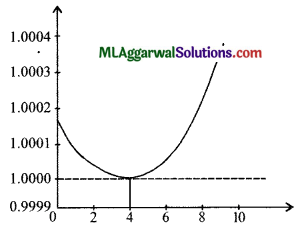Section – II
(Attempt any four questions from this section)

Question 5.
(a) Express the (i) fermi, (ii) angstrom, and (iii) micron in metre.
(b) Name the instrument you would use to measure the following :
(i) diameter of a pin
(ii) diameter of a sphere
(iii) width of a box.
(c) The main scale of a given vernier callipers is caliberated in mm. 10 divisions of vernier scale are equal to 9 divisions of the main scale. Find its least count. When the diameter of a sphere is measured, the zero of vernier scale lies between 0 and 1.1 cm and the 8th division of the vernier scale coincides with the main scale. Find the diameter of the sphere.
(i) 1 fermi (f) = 10-15 m, (ii) 1 angstrom (A) = 10_10m, (iii) 1 micron (p) = 10-6
(i) Micrometer screw gauge, (ii) vernier callipers, (iii) meter scale.
Least count (LC) of given vernier calliper = $$\frac{x}{n}$$
= $$\frac{1}{10}$$ = 0.1 mm = 0.01 cm 10
Diameter of the sphere = Main scale reading + VSD x LC
1.0 cm + 8 x 0.01 cm
= 1.08 cm.

Question 6.
(a) Obtain the relationship between S.I. and C.G.S. units of force.
(b) How long would a force of 30 N act on a body of mass 10 kg so that the body gains a velocity of 24 ms-1
(c) The velocity-time graph of a body is shown in the figure. Find its average acceleration over each of the intervals OA, AB, BC and CD.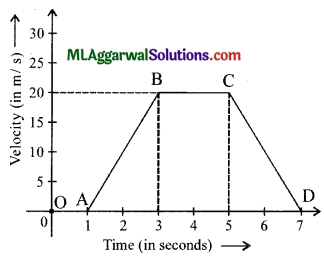(a) S.I. unit of force is newton (N) and C.G.S. unit is dyne.
1 N = 1 kg x 1 ms-2
= 1000 g x 100 cm s-2 [∵ 1 kg = 1000 g, 1m = 100 cm]
= 105 g cm s-2
∴ 1 N = 105 dyne          [ ∵ 1g cm s-2 = 1 dyne]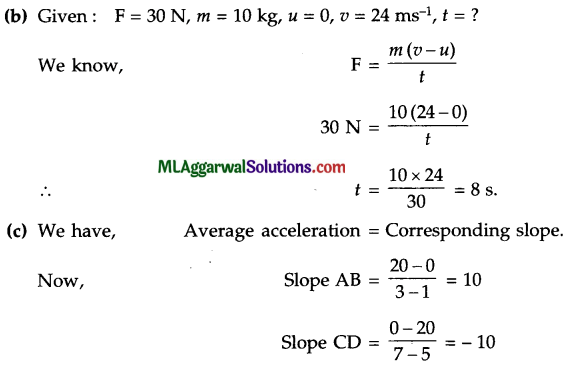The slopes of OA and BC are zero each. Thus, the average acceleration has the following values :
(1) zero over the interval OA;
(2) 10 ms-2 over interval AB;
(3) zero over interval BC; and
(4) -10 ms-2 over interval CD. (Here, the body experiences retardation)Question 7.
(a) (i) The mass of a rectangular block is 500 g. It has a base of length =10 cm and breadth = 5 cm. Calculate in S.I. unit, the average pressure exerted by the block on its base. Assume g = 10 m/s2
(ii) Should we regard the pressure at a point in a liquid as a scalar or a vector quantity?
(b) The density of the material of a solid is 5000 kg m3. Its weight in air is 0.5 kgf. What would be its apparent weight, when completely immersed in a liquid of density 8000 kg m3?
(c) Two-fifths of a cylindrical block of wood, of relative density 0.8, floats in a liquid. Calculate the density of liquid in S.I. units.
(a) (i) Weight of the block = 5 kg x 10 ms– 2 = 5 N
Its base area = 10 x 5 cm2 = 50 x 10-4 m2 = 5 x 10-3 m2
Average pressure on the base =$$\frac{5}{5 \times 10^{-3}}=10^{3} \mathrm{Nm}^{-2}$$= 1000 pascal.
(ii) The pressure at a point in a liquid is a scalar quantity.

(b) We have, Volume of solid = $$\frac{\text { Mass }}{\text { Density }}=\frac{0.5}{5 \times 10^{3}}$$= 10-4 m3
∴ Mass of liquid displaced by it = 8000 x 10-4 kg = 0.8 kg
∴ Apparent weight = Weight of solid in air – Weight of liquid displaced by it
= (0.5 – 0.8) = – 0.3 kgf
The negative sign here implies that the upthrust on the solid, when fully immersed in a denser liquid is more than the weight of the solid. Its apparent weight, in this floating condition, will then be zero.

(c) Relative density of block of wood =$$=\frac{\text { Density of block }}{\text { Density of water }}$$
Density of block = R.D. of block x Density of water
= 0.3 x 1000 kg/m3 = 800 kg/m3.
Let the volume of block be ‘V’.
∴ Volume of liquid displaced = Volume of submerged part = $$\frac{2 \mathrm{~V}}{5}$$
∴  Let the density of the liquid be ‘ρ’
Mass of displaced liquid, $$m=\left(\frac{2}{5} \mathrm{~V}\right) \rho$$
Weight of displaced liquid = mg $$=\left(\frac{2}{5} \mathrm{~V}\right) \rho g$$
According to floatation law, this is the weight of the block.
Also, Weight of the block = mg
= (Volume x Density) g
=(V x 800 kg/m3)g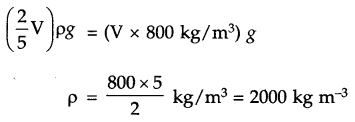Question 8.
(a) The angle between an incident ray and mirror is 50°. (i) What is the angle of incidence? (ii) What is the angle of reflection? (iii) What is the total angle formed by the ray of light?
(b) In the given figure, name the kind of mirror and show it on the diagram. Complete the ray diagram and find the position of centre of curvature and focus of the mirror if A1B1 is the image of object AB.
(c) Two plane mirrors are arranged parallel and facing each other at some separation. How many images are formed for a point source kept in between them? Show the formation of images with the help of a ray diagram.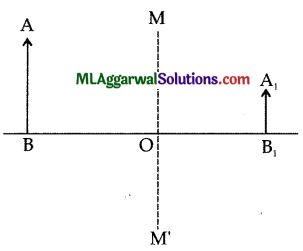(a) The ray diagram for the given situation is as shown below.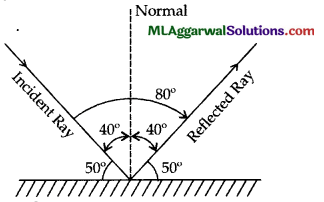(i) Angle of incidence = 40°
(ii) Angle of reflection = 40°
(iii) Total angle formed by the ray of light = 80°(b) The complete ray diagram is shown below and the mirror used is a convex mirror.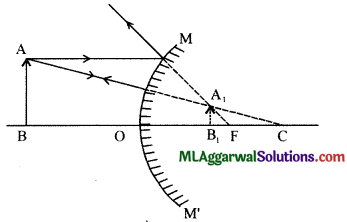(c) The number of images formed is infinite and the ray diagram is shown below :Question 9.
(a) (i) Why is sound wave called longitudinal wave ?
(ii) Will the sound be audible if the string is set into vibration on the surface of the moon ? Give reason for your answer.
(b) State three characteristics of the medium required for propagation of sound.
(c) Waves of time period 12 s have wave velocity 25 ms-1.
Find : (i) The wavelength of these waves, (ii) The horizontal distance between a wave crest and its adjoining wave trough.
(a)
(i) Sound wave is called longitudinal wave because the particles of the medium vibrate in the direction of the propagation of wave.
(ii) No, we will not hear any audible sound on the surface of the moon. This is because sound requires a medium to propagate, and there is no atmosphere on the surface of the moon. Thus, the sound will not be heard.

(b) The medium required for propagation of sound must possess the following three characteristics :
(1) The medium must be elastic.
(2) The medium must have inertia.
(3) The medium should be frictionless.

(c) Given : T = 12 s, v = 25 ms-1
(i) From relation, υ =$$\frac{\lambda}{\mathrm{T}}$$
Wavelength of wave, λ = ν x T
= 25 × 12 = 300m

(ii) The distance between a wave crest and its adjoining wave through =$$\frac{\lambda}{2}$$
$$=\frac{1}{2} \times \lambda=\frac{1}{2} \times 300=150 \mathrm{~m}$$Question 10.
(a) State three properties of a magnet.
(b) State three advantages of an electromagnet over a permanent magnet.
(c) State four properties of magnetic field lines.
(a) Three properties of a magnet are :
(1) Attractive property
(2) Directive property
(3) Poles exist in pairs.

(b) An electromagnet has the following advantages over a permanent magnet:
(1) The polarity of an electromagnet can be reversed by reversing the direction of current in its solenoid.
(2) An electromagnet can produce a strong magnetic field.
(3) The strength of the magnetic field of an electromagnet can be easily changed by changing the current (or the number of turns) in its solenoid.

(c) Magnetic field lines must have the following four properties :
(1) They travel from the north to the south pole of a magnet outside the magnet and from south to the north inside the magnet.
(2) They are closed and continuous curves.
(3) The tangent drawn at any point of the magnetic lines of force represents the direction of the magnetic field at that point.
(4) Two magnetic fields do not intersect each other.